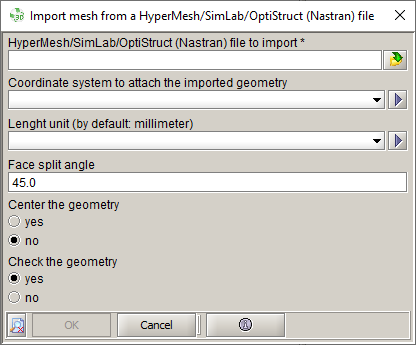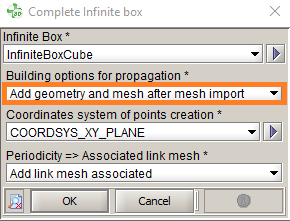# Flux mesh import workflow

## Introduction

This section presents the steps to follow in Flux to import the mesh through the Nastran file.

## Workflow

In the following table, the steps to follow in Flux for a nominal scenario are presented:
• Open Flux, out of the Modeler context
1. Import the mesh:

Project > Import > Import mesh > Import mesh from a HyperMesh/SimLab/OptiStruct (Nastran) file

• Select the Nastran (or OptiStruct) file to import
• Define the coordinate system and units if necessary
• Change the Face split angle if necessary: this angle corresponds to the minimal angle of facets* separation (two facets defining an angle superior or equal to this value will be separated to two faces. For an inferior angle, the facets are merged)
• Center or not the geometry
• Verify or not the geometryThat allows doing a "fast & quick" check of the geometry defaults: superimposed points, intersections between lines. To make a more complete check, launch the command:

Geometry > Check geometry

After the import, we recover in Flux:
• The mesh elements
• A "mesh object" containing the imported mesh
• The lines, faces from the facets merge and the volumes from SimLab/HyperMesh components
• The volume regions from the components

* A facet corresponds to an elementary face defined by a mesh surface element

2. It is possible to create the infinite box in Flux after mesh import (if air box has not been created in SimLab or HyperMesh):
• Create infinite box:

Physics > Infinite box > New

• Complete infinite box to generate the air volume and the associated mesh:

Physics > Infinite box > Complete infinite box

Choose the option:

Add geometry and mesh after mesh import: